login  home  contents  what's new  discussion  bug reports help  links  subscribe  changes  refresh  edit

# Edit detail for numerical linear algebra revision 4 of 5

 1 2 3 4 5 Editor: test1 Time: 2014/10/07 13:56:49 GMT+0 Note:

changed:
-solve(rhs(eigen.1),15)
solve(rhs(eigen.1),10.0^(-15))

changed:
-Why would you expect to be able to recover the characteristic polynomial?  There is always round off error for finite precision arithmetic. For integer approximations, it would be worse.
-The command {\tt solve: (Polynomial Fraction Integer, PositiveInteger)->List Equation Polynomial Integer}
-solves the equation over the integers, so it is {\it not} accurate. For example:
To recover characteristic polynomial one needs good precision, which means very
small second argument to solve.  Giving large second arguments means very poor
accuracy:

removed:
-ev:= solve(rhs(eigen.1),1.0*10^(-50))
-cp:= reduce(*, [rhs(x)-lhs(x) for x in ev])

To have use of precise solution we also need to increase precision of other
floating point computations using digits.  Unfortunately, this leads to
ugly display, so we only show final result:
\begin{axiom}
digits(120)
ev:= solve(rhs(eigen.1),1.0*10^(-100));
cp:= reduce(*, [rhs(x)-lhs(x) for x in ev]);
rhs(eigen.1) - cp
\end{axiom}


I'm new to Axiom, so maybe I'm doing things in a stupid way.

I want to get (estimates of) the eigenvalues of a 10x10 matrix of floats:

fricas
m := matrix([[random()$Integer for i in 1..10] for j in 1..10]); sm := m + transpose(m); smf:Matrix Float := sm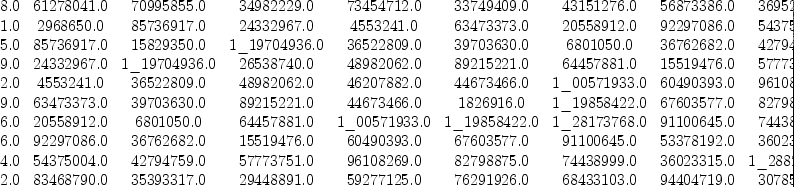(1) Type: Matrix(Float) The problem is: If I now call eigenvalues(smf) on the symmetric float matrix smf Axiom 3.0 Beta (February 2005) runs for a very long time (uncomment code if you want to try it): fricas )set messages time on Try this:: fricas eigen:=eigenvalues(sm)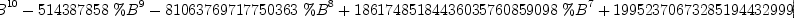(2) Type: List(Union(Fraction(Polynomial(Integer)),SuchThat?(Symbol,Polynomial(Integer)))) fricas Time: 0.01 (IN) + 0.01 (EV) + 0.07 (OT) = 0.09 sec solve(rhs(eigen.1),10.0^(-15))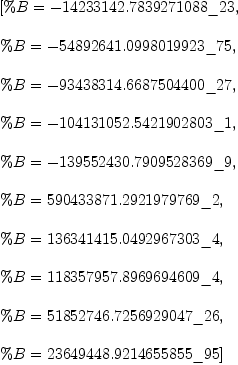(3) Type: List(Equation(Polynomial(Float))) fricas Time: 0.03 (EV) + 0.01 (OT) = 0.04 sec Thank you! This helps, but doesn't answer everything. Since interestingly: fricas charpol := reduce(*, [ rhs(x) - lhs(x) for x in % ])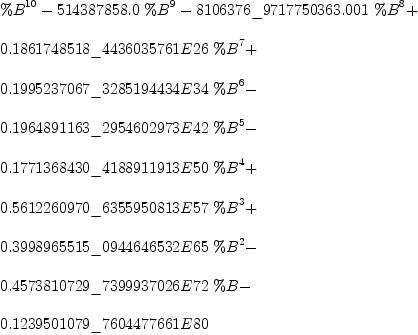(4) Type: Polynomial(Float) fricas Time: 0 sec we cannot recover the characteristic polynomial from this solution: Even if a large number is passed to solve, accuracy does not increase. To recover characteristic polynomial one needs good precision, which means very small second argument to solve. Giving large second arguments means very poor accuracy: fricas solve(x+11/10,3)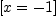(5) Type: List(Equation(Polynomial(Fraction(Integer)))) fricas Time: 0.01 (IN) = 0.01 sec To have use of precise solution we also need to increase precision of other floating point computations using digits. Unfortunately, this leads to ugly display, so we only show final result: fricas digits(120)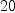(6) Type: PositiveInteger? fricas Time: 0 sec ev:= solve(rhs(eigen.1),1.0*10^(-100)); Type: List(Equation(Polynomial(Float))) fricas Time: 0.09 (EV) = 0.09 sec cp:= reduce(*, [rhs(x)-lhs(x) for x in ev]); Type: Polynomial(Float) fricas Time: 0 sec rhs(eigen.1) - cp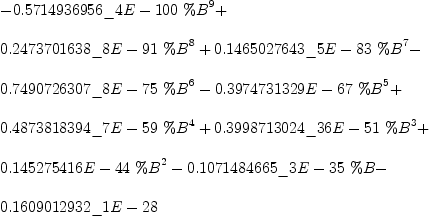(7) Type: Polynomial(Float) fricas Time: 0 sec fricas A:=[[a,b],[c,d]]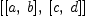(8) Type: List(List(Symbol)) fricas Time: 0.01 (OT) = 0.01 sec fricas A:=matrix[[cos(x),-sin(x)],[sin(x),cos(x)]]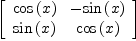(9) Type: Matrix(Expression(Integer)) fricas Time: 0.04 (OT) = 0.04 sec A(1,1)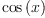(10) Type: Expression(Integer) fricas Time: 0 sec eigen:=eigenvalues(A) There are 1 exposed and 1 unexposed library operations named eigenvalues having 1 argument(s) but none was determined to be applicable. Use HyperDoc Browse, or issue )display op eigenvalues to learn more about the available operations. Perhaps package-calling the operation or using coercions on the arguments will allow you to apply the operation. Cannot find a definition or applicable library operation named eigenvalues with argument type(s) Matrix(Expression(Integer)) Perhaps you should use "@" to indicate the required return type, or "$" to specify which version of the function you need.

Unfortunately, currently eigenvalues does not work for general expressions, which causes the failure above.

fricas
A:=matrix[[cos(x)-L,-sin(x)],[sin(x),cos(x)-L]]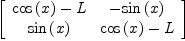(11)
Type: Matrix(Expression(Integer))
fricas
Time: 0 sec
A(1,1)*A(2,2)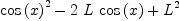(12)
Type: Expression(Integer)
fricas
Time: 0 sec
A(2,1)*A(1,2)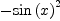(13)
Type: Expression(Integer)
fricas
Time: 0 sec
A(1,1)*A(2,2)-A(2,1)*A(1,2)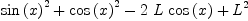(14)
Type: Expression(Integer)
fricas
Time: 0 sec
B := solve(A(1,1)*A(2,2)-A(2,1)*A(1,2)=0,L)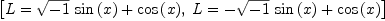(15)
Type: List(Equation(Expression(Integer)))
fricas
Time: 0.01 (IN) + 0.01 (EV) + 0.01 (OT) = 0.03 sec
B(1)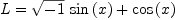(16)
Type: Equation(Expression(Integer))
fricas
Time: 0 sec

fricas
solve(x^2 - 2,x)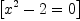(17)
Type: List(Equation(Fraction(Polynomial(Integer))))
fricas
Time: 0.01 (EV) = 0.01 sec
sqrt(2)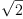(18)
Type: AlgebraicNumber?
fricas
Time: 0 sec
solve(x^2=4,x)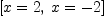(19)
Type: List(Equation(Fraction(Polynomial(Integer))))
fricas
Time: 0.01 (IN) = 0.01 sec

fricas
P:=matrix[[a, b], [1.0 - a, 1.0 - b]]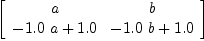(20)
Type: Matrix(Polynomial(Float))
fricas
Time: 0 sec
eigenvectors(P)
Internal Error
The function eigenvectors with signature hashcode is missing from
domain EigenPackage(Float)